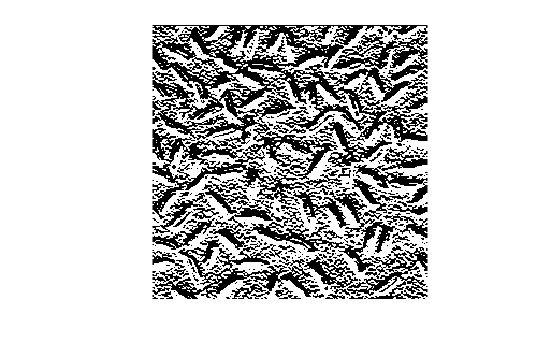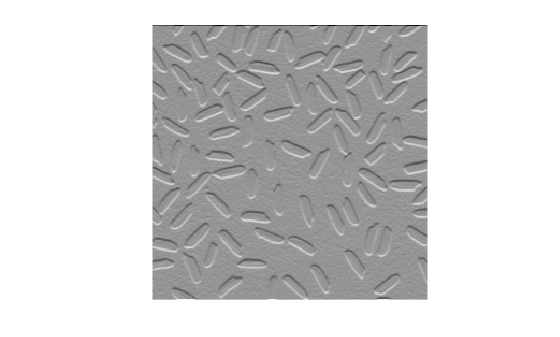# mat2gray

Convert matrix to grayscale image

## Syntax

``I = mat2gray(A,[amin amax])``
``I = mat2gray(A)``

## Description

````I = mat2gray(A,[amin amax])` converts the matrix `A` to a grayscale image `I` that contains values in the range 0 (black) to 1 (white). `amin` and `amax` are the values in `A` that correspond to 0 and 1 in `I`. Values less than `amin` are clipped to 0, and values greater than `amax` are clipped to 1.```

example

````I = mat2gray(A)` sets the values of `amin` and `amax` to the minimum and maximum values in `A`.```

## Examples

collapse all

Read an image and display it.

```I = imread('rice.png'); figure imshow(I)```Perform an operation that returns a numeric matrix. This operation looks for edges.

```J = filter2(fspecial('sobel'),I); min_matrix = min(J(:))```
```min_matrix = -779 ```
`max_matrix = max(J(:))`
```max_matrix = 560 ```

Note that the matrix has data type `double` with values outside of the range [0,1], including negative values.

Display the result of the operation. Because the data range of the matrix is outside the default display range of `imshow`, every pixel with a positive value displays as white, and every pixel with a negative or zero value displays as black. It is challenging to see the edges of the grains of rice.

```figure imshow(J)```Convert the matrix into an image. Display the maximum and minimum values of the image.

```K = mat2gray(J); min_image = min(K(:))```
```min_image = 0 ```
`max_image = max(K(:))`
```max_image = 1 ```

Note that values are still data type `double`, but that all values are in the range [0, 1].

Display the result of the conversion. Pixels show a range of grayscale colors, which makes the location of the edges more apparent.

```figure imshow(K)```## Input Arguments

collapse all

Input image, specified as a numeric matrix.

Input black and white values, specified as a 2-element numeric vector.

• Values in input image `A` that are less than or equal to `amin` are mapped to the value 0 in the intensity image, `I`.

• Values in `A` that are greater than or equal to `amax` are mapped to the value 1 in `I`.

## Output Arguments

collapse all

Output intensity image, returned as a numeric matrix with values in the range [0, 1].

Data Types: `double`

## Version History

Introduced before R2006a